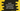# Find the smallest of n values using min() in Swift## Swift min() function explanation with example:

min() function in Swift can be used to find the smallest value among n different values. This method can be used to find the smallest among n provided elements.

### Definition:

min() is defined as below:

``func min<T>(_ a: T, _ b: T, _ c: T, _ rest: T...) -> T``

Where,

• T is Comparable
• a is the first value to compare
• b is the second value to compare
• c is the third value to compare
• rest is zero or more values to compare

The return value of this function is the least of all provided values.

If there are more than one equal values as the smallest, it returns the first value found.

### Example program:

Let’s take a look at the below program:

``````print(min(1, 2))
print(min(1, 2, 3))
print(min(1, 2, 3, 4, 5, 6, 7, 8, 9, 0))``````

It is finding the smallest value for two, three and ten values. If you run it, it will print the below output:

``````1
1
0``````

### Example with custom objects:

We can also use custom objects with min() function. For that, we need the class as subclass of Comparable. For example:

``````class Student: Comparable{
var name: String
var age: Int

init(name: String, age: Int) {
self.name = name
self.age = age
}

static func < (lhs: Student, rhs: Student) -> Bool {
return lhs.age < rhs.age
}

static func == (lhs: Student, rhs: Student) -> Bool {
return lhs.age == rhs.age
}

func toString(){
print("Name: \(self.name), Age: \(self.age)")
}
}

let alex = Student(name: "Alex", age: 20)
let bob = Student(name: "Bob", age: 18)
let eli = Student(name: "Eli", age: 17)

min(alex, bob).toString()
min(eli, bob).toString()
min(alex, bob, eli).toString()``````

Here,

• Class Student is a subclass of Comparable.
• It implements the < function and == function.
• We created three objects of this class, alex, bob and eli and used these objects with min. The toString function prints the Name and Age of the Student object returned by the min function.

If you run this program, it will print the below output:

``````Name: Bob, Age: 18
Name: Eli, Age: 17
Name: Eli, Age: 17``````